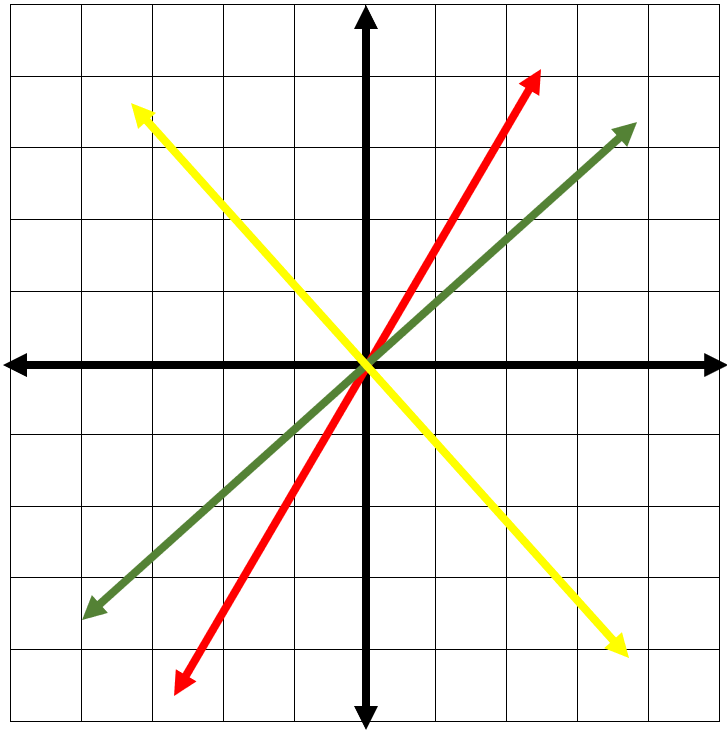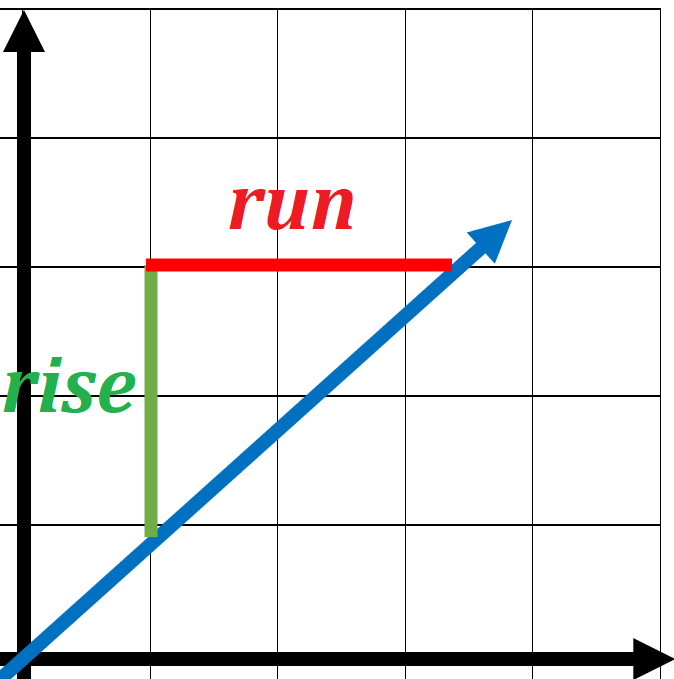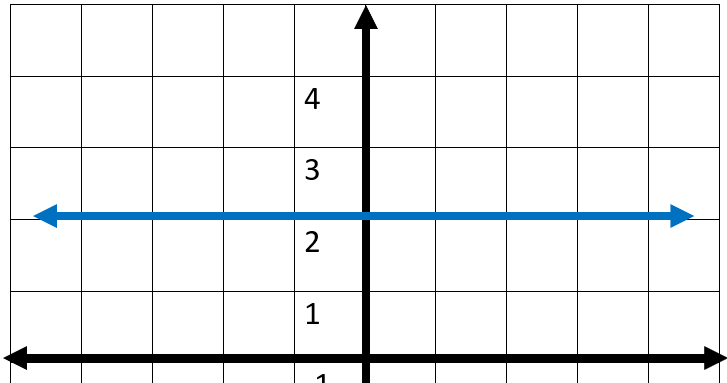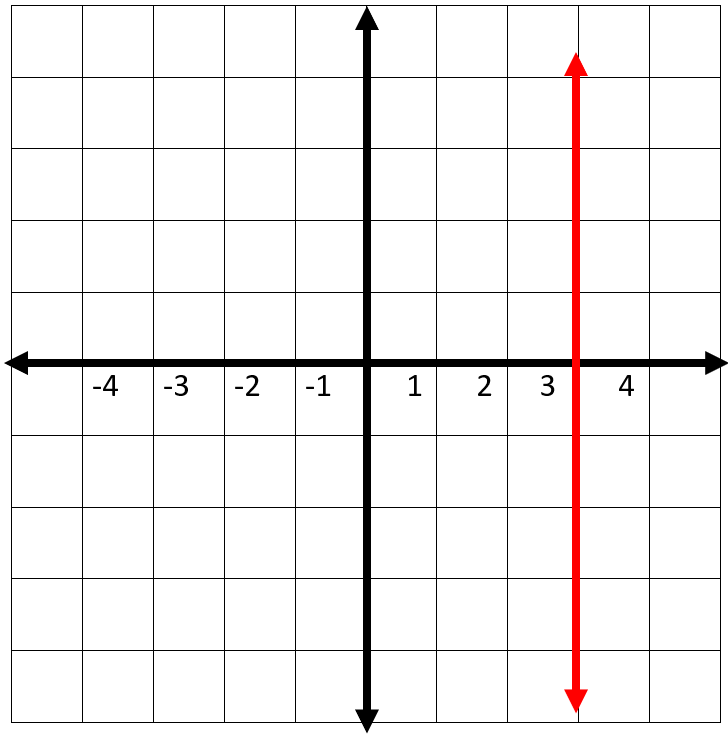## How to find the slope of a line?

The notion of slope is something you find many times in normal life. Consider pushing a wagon down some ramp or climbing up stairs. The stairs and ramp both have a slope. It’s possible to describe that slope, or the item’s steepness by considering vertical as well as horizontal movement along them. When we speak, words such as “gradual” or “steep” are used for describing slope. Along a gradual slope, the majority of movement will be horizontal. Alongside a steep slope, vertical movement is greater.

### Defining Slope

The mathematical definition of slope is quite similar to our everyday one. With math, slope is utilized for describing the steepness and direction of the lines. Via merely looking at the graph of a line, it’s possible to learn things about its slope, particularly in relation to other lines graphed on the exact same coordinate plane. Think about the graphs of the 3 lines below:Firstly, study green and red lines green and red. If one thinks of the lines as hills, they’d say the red line is steeper than the green line. the red line has a greater slope than the green line.

After that, see that green line and red line slant upward as one moves from left to right. You could say that these 2 lines have a positive slope. The yellow line slants downward from left to right. The yellow line has a negative slope. Utilizing 2 of the points on the line, it’s possible to find the line’s slope via discovering the rise and the run. The vertical change between 2 points is known as a rise, and the horizontal change is known as a run. The slope equals the rise divided by the run: $$slope \ = \ \frac{rise}{run}$$### Discovering the Slope of a Line if Given Two Points

We have seen that it’s possible to discover a line’s slope on a graph via measuring its rise and the run. It is also possible to discover the slope of a straight line without a graph if one knows the coordinates of any two points on that line. Every point has a set of coordinates: an $$x$$-value and a $$y$$-value, written as an ordered pair $$(x, y)$$. The $$x$$ value informs one where a point is horizontally. The $$y$$ value informs one where the point is vertically.
Think about 2 points on a line, Point $$1$$ and Point $$2$$. Point $$1$$ has coordinates $$(x_{1}, y_{1})$$ and Point $$2$$ has coordinates $$(x_{2}, y_{2})$$.
Its rise is the vertical distance between the $$2$$ points, which is also the difference between their $$y$$-coordinates. That makes the rise $$y_{2} \ − \ y_{1}$$. The run in between these $$2$$ points is the difference in the $$x$$-coordinates, or $$x_{2} \ − \ x_{1}$$.

So, $$slope \ = \ \frac{rise}{run} \ \$$ or $$\ m \ = \ \frac{y_{2} \ − \ y_{1}}{x_{2} \ − \ x_{1}}$$

### Discovering the Slopes of Horizontal and Vertical Lines

Up to now, we’ve considered lines which run “uphill” or “downhill.” Their slopes might be either steep or gradual, however, they’re always going to be negative or positive numbers. However, there are 2 additional types of lines, vertical and horizontal. What’s the slope of a flat line or level ground? Or of a wall or a vertical line?

So, think about a horizontal line on a graph. It doesn’t matter which two points are picked on the line, they’ll forever have the same y-coordinate. The equation for this line is $$y \ = \ 2$$. The equation additional can be written as $$y \ = \ (0)x \ + \ 2$$.Utilizing the form $$y \ = \ (0)x \ + \ 2$$, one will see the slope is $$0$$. One can additionally utilize the slope formula with 2 points on this horizontal line to determine the slope of this horizontal line. Utilizing $$(1, 2)$$ as Point $$1$$ and $$(2, 2)$$ as Point $$2$$, one gets:
$$m \ = \ \frac{y_{2} \ − \ y_{1}}{x_{2} \ − \ x_{1}} \ = \ \frac{2 \ − \ 2}{2 \ − \ 1}$$
The slope of this horizontal line is $$0$$.

So, think about any horizontal line. It doesn’t which 2 points on the line are picked, they’ll forever have the same $$y$$-coordinate. Therefore, whenever one applies the slope formula, the numerator is always going to end up as $$0$$. Zero divided by any non-zero number is $$0$$, therefore, the slope of any horizontal line is forever $$0$$.

What about vertical lines? In this case, it doesn’t matter what 2 points are picked, they’ll forever have the same x-coordinate. The equation for this line is $$x \ = \ 3$$.There’s no way this equation can be put into the slope-point form since the coefficient of $$y$$ is $$0$$ $$(x \ = \ 0y \ + \ 3)$$.

Therefore, what occurs whenever one uses the slope formula with 2 points on this vertical line to determine the slope? Utilizing $$(3,2)$$ as Point $$1$$ and $$(3,-1)$$ as Point $$2$$, one comes up with:
$$m \ = \ \frac{y_{2} \ − \ y_{1}}{x_{2} \ − \ x_{1}} \ = \ \frac{-1 \ − \ 2}{3 \ − \ 3}$$
However, dividing by zero doesn’t have a meaning for the set of real numbers. Due to that fact, it’s said the slope of this vertical line is undefined. That’s true for all vertical lines. they will all have an undefined slope.

### Exercises for Finding Slope

1) $$y = 4x - 4$$$$\ \Rightarrow \$$

2)  $$2y = -6x - 3$$$$\ \Rightarrow \$$

3) $$30x + 6 - 3y = 0$$$$\ \Rightarrow \$$

4) $$9x + 3 - 3y = 0$$$$\ \Rightarrow \$$

5) $$-10x + 5 - 2y = 0$$$$\ \Rightarrow \$$

6) Find the slope of the line passing through $$(4, 1),(0, -75)$$$$\ \Rightarrow \$$

7) $$y = -3x - 5$$$$\ \Rightarrow \$$

8) Find the slope of the line passing through $$(2, 7),(5, 43)$$$$\ \Rightarrow \$$

9) Find the slope of the line passing through $$(4, 1),(3, 4)$$$$\ \Rightarrow \$$

10) Find the slope of the line passing through $$(0, 0),(-3, 30)$$$$\ \Rightarrow \$$

1) $$y = 4x - 4$$$$\ \Rightarrow \$$Slope$$\color{red}{ \ =4}$$
2) $$2y = -6x - 3$$$$\ \Rightarrow \ \color{red}{y= \frac{-6}{2}x - \frac{3}{2} }$$$$\ \Rightarrow \$$Slope$$\ \color{red}{=\frac{-6}{2} \ =-3}$$
3) $$30x + 6 - 3y = 0$$$$\ \Rightarrow \ \color{red}{y= \frac{30}{3}x - \frac{6}{3} }$$$$\ \Rightarrow \$$Slope$$\ \color{red}{=\frac{30}{3} \ =10}$$
4) $$9x + 3 - 3y = 0$$$$\ \Rightarrow \ \color{red}{y= \frac{9}{3}x - \frac{3}{3} }$$$$\ \Rightarrow \$$Slope$$\ \color{red}{=\frac{9}{3} \ =3}$$
5) $$-10x + 5 - 2y = 0$$$$\ \Rightarrow \ \color{red}{y= \frac{-10}{2}x - \frac{5}{2} }$$$$\ \Rightarrow \$$Slope$$\ \color{red}{=\frac{-10}{2} \ =-5}$$
6) Find the slope of the line passing through $$(4, 1),(0, -75)$$$$\ \Rightarrow \ \color{red}{\frac{-75 \ - \ 1}{0 \ - \ 4} = }$$$$\color{red}{ \ \frac{-76}{-4} = 19}$$
7) $$y = -3x - 5$$$$\ \Rightarrow \$$Slope$$\color{red}{ \ =-3}$$
8) Find the slope of the line passing through $$(2, 7),(5, 43)$$$$\ \Rightarrow \ \color{red}{\frac{43 \ - \ 7}{5 \ - \ 2} = }$$$$\color{red}{ \ \frac{36}{3} = 12}$$
9) Find the slope of the line passing through $$(4, 1),(3, 4)$$$$\ \Rightarrow \ \color{red}{\frac{4 \ - \ 1}{3 \ - \ 4} = }$$$$\color{red}{ \ \frac{3}{-1} = -3}$$
10) Find the slope of the line passing through $$(0, 0),(-3, 30)$$$$\ \Rightarrow \ \ \color{red}{\frac{30 \ - \ 0}{-3 \ - \ 0} = }$$$$\color{red}{ \ \frac{30}{-3} = -10}$$

## Finding Slope Practice Quiz

### The Most Comprehensive FTCE Math Preparation Bundle

$76.99$34.99

### The Most Comprehensive HiSET Math Preparation Bundle

$76.99$36.99

### FSA Grade 6 Math Prep 2020

$19.99$14.99

### HiSET Math for Beginners

$24.99$14.99